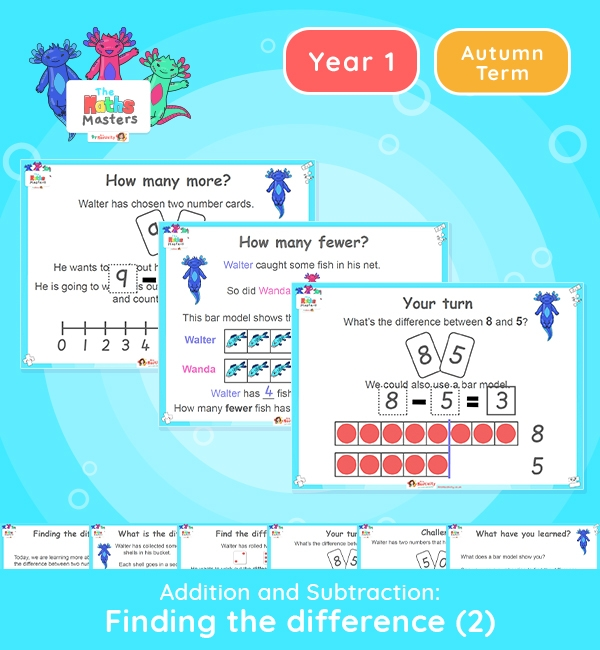# Year 1 | Subtracting by Finding the Difference Part 2 Lesson Presentation## Year 1 addition and subtraction resources

Aligned with the maths mastery approach, this Year 1 | Subtracting by Finding the Difference Part 2 Lesson Presentation is fully editable and designed for the Year 1 maths curriculum to cover the following curriculum objectives:

This is the second of two lessons on finding the difference. It focuses on the use of bar models and number lines to work out the difference between two numbers.

Small Steps: Subtraction – finding the difference

NC Links: Read, write and interpret mathematical statements involving addition (+), subtraction (-), and equals (=) signs. • Add and subtract one digit numbers to 10, including zero. • Solve one step problems that involve addition and subtraction using concrete objects and pictorial representations.

Ready-to-progress criteria:  1NF-1 Develop fluency in addition and subtraction facts within 10

Previous experience: Begin to experience partitioning and combining numbers within 10

1AS-2 Read, write and interpret equations continuing addition, subtraction  and equals symbols, and relate additive expressions and equations to real-life contexts.

Previous Experience: Devise and record number stories, using pictures, numbers and symbols.

TAF Statements: Working Towards Add and subtract (one digit numbers) explaining their method verbally in pictures or using apparatus.

Working At: Recall all the number bonds to and within 10 and use these to reason with.

Explore our other year 1 addition and subtraction resources.

## Recently Viewed